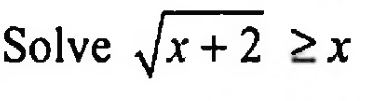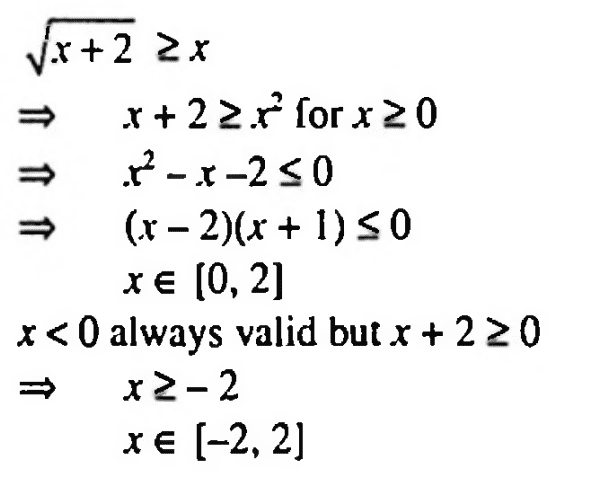# An inequality involving ##x## on both sides: ##\sqrt{x+2}\ge x##

• brotherbobby

#### brotherbobby

Homework Statement
Solve : ##\sqrt{x+2} \ge x##
Relevant Equations
For ##\sqrt{f(x)}##, we must have both (1) ##\sqrt{f(x)} \ge 0## and (2) ##f(x)\ge 0##Problem statement :
Let me copy and paste the problem as it appears in the text on the right.

Attempt (myself) : By looking at ##\large{\sqrt{x+2}\ge x}##, from my Relevant Equations above, we have the following :

1. Outcome ##\mathbf{x \ge 0}##, since square roots are always positive.
2. Function inside the square root, ##x+2 \ge 0\Rightarrow \mathbf{x \ge -2}##.

Armed with these inequalities, I square both sides of it. ##x+2 \ge x^2\Rightarrow x^2 - x - 2 \le 0\Rightarrow (x-2)(x+1)\le 0\Rightarrow \mathbf{-1\le x \le 2}##.

Looking at the three (bold faced) solutions above and merging them, I find that the answer must be : ##\boxed{\mathbf{0 \le x \le 2\Rightarrow x \in [0,2]}}##.

But the book has a different answer.

Attempt (Text Solution) : I copy and paste below the solution to the problem as it appears in the text.Doubt : Of course is the text correct. For instance how can ##x## lie in the interval ##[-2,2]##? That would imply that ##x## can be equal to -1, which would make the square root of a quantity negative.

A hint or suggestion would be welcome as to where have I gone wrong.

•nuuskur and Delta2
brotherbobby said:
1. Outcome ##\mathbf{x \ge 0}##, since square roots are always positive.

It is indeed the case that $\sqrt{x + 2} \geq 0$ for $x \geq - 2$, but this admits the possibility that $$\sqrt{x + 2} \geq 0 \geq x.$$ Thus $x \in [-2,0]$ satisfies the inequality.

••SammyS, Mark44 and Delta2
Different approach:

## \sqrt { x + 2 } = t ##
## x = t ^ 2 - 2 ##
## t \geq t ^ 2 - 2 ##
## t ^ 2 - t - 2 \leq 0 ##
## t \in [-1, 2] ## and because ## t \geq 0 ##
## t \in [0, 2] ##
## \sqrt { x + 2 } \geq 0 ## and ## \sqrt { x + 2 } \leq 2 ##
## x + 2 \geq 0 ## and ## x + 2 \leq 4 ##
## x \geq -2 ## and ## x \leq 2 ##
## x \in [-2, 2] ##

pasmith said:
Thus $x \in [-2,0]$ satisfies the inequality.
As does the interval [0, 2], which I believe you knew but didn't state.

If you graph ##y = \sqrt{x + 2}## and ##y = x## on the same coordinate axis system, you can see that ##y = \sqrt{x + 2}## lies above the graph of y = x for ##x \in [-2, 2)## and meets it at x = 2.

•Gavran and e_jane